Share filter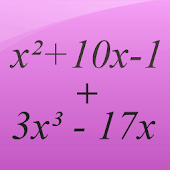Developed by in Education category

4/5.0 Ratings

10,000 - 50,000 Installs

303 Reviews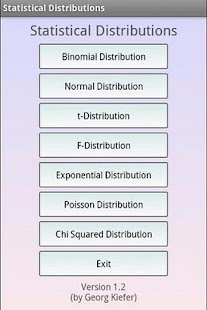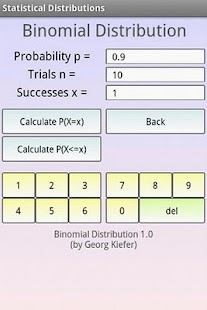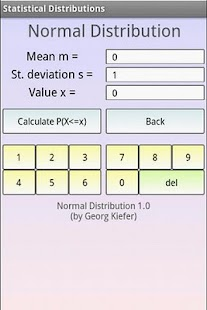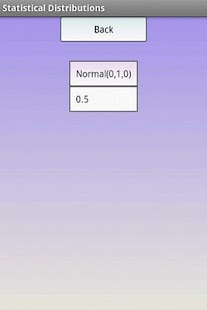Description

This free math app is a statistical calculator which calculates the values of different Statistical Distributions.
The following distributions are available:

- Binomial Distribution
- Normal Distribution
- Students t-Distribution
- F-Distribution
- Exponential Distribution
- Poisson Distribution
- Chi Squared Distribution

Best mathematical tool for school and college! If you are a student, it will helps you to learn statistics and probability theory.

Note: Statistics is the study of the collection, organization, analysis, interpretation and presentation of data.
Probability theory is the branch of mathematics concerned with probability, the analysis of random phenomena. The central objects of probability theory are random variables, stochastic processes, and events.

This content has been crawled through Play Store, we don't store any APK file.